February 16, 2016

## Content

• GEO
• Entity - Relationship Models
• Accessing GEO from R
• Exercises with R
• Desired difficulty
• Error minimization

## What is GEO?

It is an international public repository that archives and distributes gene expression data submitted by the research community

• microarray,
• next-generation sequencing,
• and other forms of high-throughput functional genomics data

## MIAME

### Minimum Information About a Microarray Experiment

The minimum information that should be included when describing a microarray experiment.

Many journals and funding agencies require microarray data to comply with MIAME.

GEO encourage submitters to supply MIAME compliant data.

## Critical elements in MIAME

### with examples

• The raw data for each hybridization
• CEL or GPR files
• The final normalized data for the set of hybridizations
• the gene expression data matrix used to draw the conclusions from the study
• The essential sample annotation including experimental factors and their values
• compound and dose in a dose response experiment

Homework: Write down the new words or concepts and Google them

## Critical elements in MIAME (cont)

• which raw data file relates to which sample,
• which samples are technical/biological replicates
• Sufficient annotation of the array
• gene identifiers, genomic coordinates,
• probe oligonucleotide sequences
• reference commercial array catalog number
• The essential laboratory and data processing protocols
• what normalization method was used

## What is the Structure of GEO?

• Each author submits a series that contains one or more samples
• Each sample uses a platform to investigate one or more organisms
• Each platform has several probes to represent genes

All these entities are related to each other

## Entity-Relationship modeling

GEO is an example of a pattern that is important in Data Science:

Data can have a structure that help us to manage and understand it

• The most common database systems are relational databases
• They store entities and relationships between them

## Entity-Relationship modeling

• Entities: objects, nouns
• They exist independently of others
• They may have attributes
• Relationships: verbs
• connect two or more entities
• They may have attributes

## Linguistic analysis

• Common noun: entity type, class
• proper noun: entity, instance
• verb: relationship

Eg. The genes of an organism have expression levels that depending on the growth medium

## Example

The genes of an organism have expression levels that depending on the growth medium

Entities and their attributes

• genes: name, location
• organisms: scientific name
• growth medium: concentration, temperature

Relationships and attributes

• each gene is expressed under a growth medium
• the expression has a level

## Cardinality

It is important to declare the possible number of relationships for each entity instance

For a given relationship between entities A and B

• Are all instances in A related to at least one instance of B?
• Can any instance of A be related to several instances of B?

Technical names: Surjective and Injective relationships. Check them

## Cardinality example

The genes of an organism have expression levels that depending on the growth medium

• one organism has one or more genes
• each gene belongs to one and only one organism
• each gene has only one expression level on each growth medium

## Accession Keys or Identifiers

Each instance of an entity should have an attribute to identify it

• Think of a “T.C. Kimlik No” of the entity
• One and only one for each instance
• Not shared with another instance
• Stable: never changes

Is “given name” a good identifier for a person?

## Visualization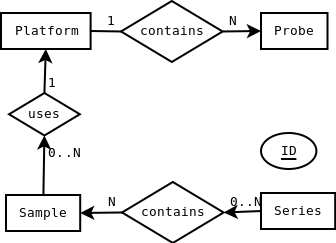## Alternative Visualization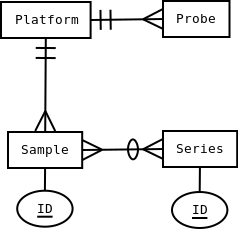## Crow’s foot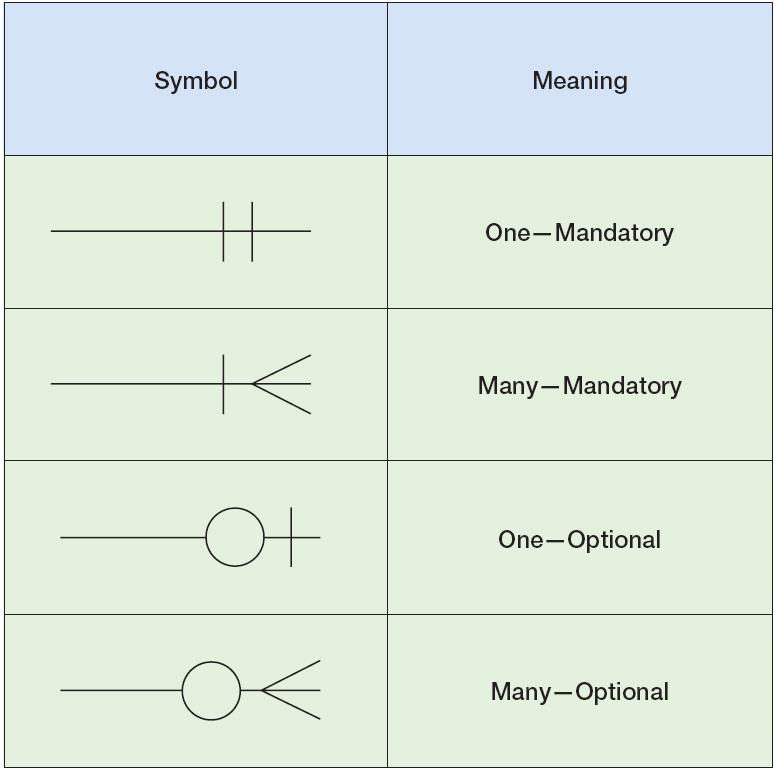## Homework

Identify the entities and relationships on the following cases.

Draw E-R diagrams for each of them

• You as student of Istanbul University
• Human cells
• Streets of Istanbul

## GEO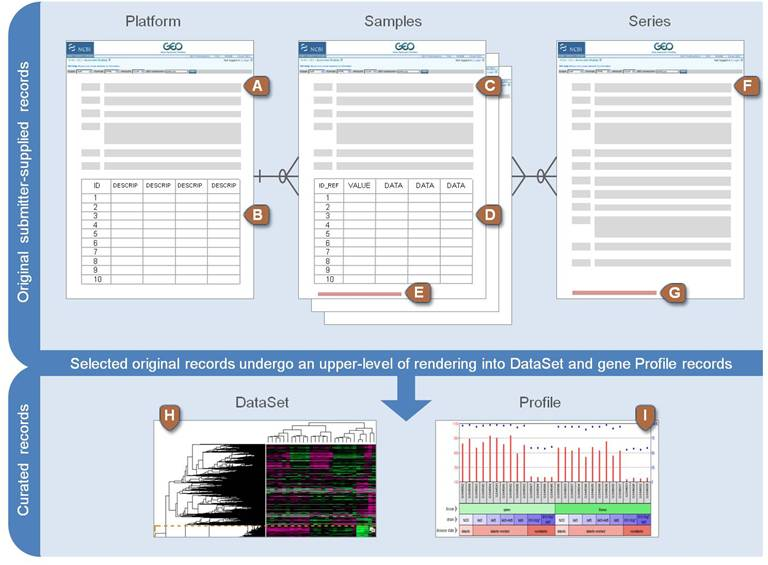## Platform records are supplied by submitters

A Platform record is composed of a summary description of the array or sequencer and, for array-based Platforms, a data table defining the array template.

• Text description of the array or sequencer
• Text tab-delimited table of the array template

Each Platform record is assigned a unique and stable GEO accession number (GPLxxx).

A Platform may reference many Samples that have been submitted by multiple submitters.

Example Platform record »

## Samples are supplied by submitters

Describe how a single sample was handled, the manipulations it underwent, and the abundance measurement of each element derived from it.

Each Sample record is assigned a unique and stable GEO accession number (GSMxxx).

A Sample entity must reference only one Platform and may be included in multiple Series.

• Text description of the biological sample and protocols to which it was subjected
• Text tab-delimited table of processed hybridization result
• Original raw data file, or processed sequence data file

Example Sample record »

## Series are supplied by submitters

A Series record links together a group of related Samples and provides a focal point and description of the whole study.

• Text description of the overall experiment
• Tar archive of original raw data files, or processed sequence data files

Series records may also contain tables describing extracted data, summary conclusions, or analyses.

Each Series record is assigned a unique and stable GEO accession number (GSExxx).

Example Series record »

## DataSet are made by GEO curators

• A curated collection of biologically and statistically comparable GEO Samples
• forms the basis of GEO’s suite of data display and analysis tools.
• Samples within a DataSet refer to the same Platform
• they share a common set of array elements
• Value measurements for each Sample within a DataSet are assumed to be calculated in an equivalent manner
• considerations such as background processing and normalization are consistent across the DataSet.
• Information reflecting experimental factors is provided through DataSet subsets.

Example DataSet record »

## How do we read GEO data into R?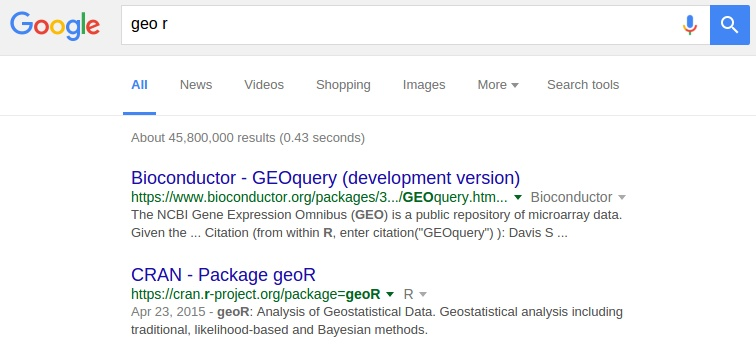Notice that the second answer is not related to us

## R packages

Remember that R can be extended with packages

• When needed we load into the session using library(package)

Anybody can make new packages. Most of them are found in a repository

• CRAN
• Bioconductor

## Bioconductor

A set of libraries used to analyze molecular biology results

GEOquery is a package found in Bioconductor

## The getGEO function

getGEO(GEO = NULL, filename = NULL, destdir = tempdir(), GSElimits=NULL,
GSEMatrix=TRUE, AnnotGPL=FALSE, getGPL=TRUE)
• GEO: A character string representing a GEO object for download and parsing.
• eg. GDS2225, GSE3541, GSM81022, GPL341
• filename: The filename of a previously downloaded GEO SOFT format file.
• Either one of GEO or filename may be specified, not both.
• destdir: The destination directory for any downloads. Defaults to a temporal dir.
• You may want to specify a different directory if you want to save the file for later use.
• Doing so is a good idea if you have a slow connection, as some of the GEO files are HUGE!

## Homework

### Reading microarray data from GEO

• Always use destdir parameter to avoid excessive Internet usage
• use RStudio Environment pane to examine the components of the resulting object
• which are the samples?
• which is the platform?
• which are the genes?

Practice at home. We will need this next week

## Desired difficulty

A situation which

• makes something harder to learn initially
• harder to “encode”
• but makes it easier to recall and apply later
• easier to “retrieve”

“That a learning difficulty can be desirable in the long run is counterintuitive; students and instructors typically conflate immediate performance with longterm learning and therefore strive to avoid impediments to performance.”

Bjork Learning & Forgetting Lab

## Kolay Gelsin?

Many studies whow that doing things the easy way results in bad results

It is a good idea for manual and repetitive work

It is a very bad idea for creative and intellectual work

Remember: Thinking is like the gym

• It is hard if you don’t practice
• We are lazy and we avoid it
• We improve with practice
• Then we like it. And we look better

## Error minimization

In statistics the word “error” does not mean “mistake”.

• Mistake is something we did wrong
• Error is the lack of precision even when we did everything right

Mistakes are about us. Errors are the difference between “models” and “reality”.

## Errors in data summarization

If we have a vector x and we want to find a single value y to “represent” it, how do we choose y?

Since usually all x[i] are different, there will be an error if we represent them with a single y.

The best y will be the one which has the lowest error.

How do we measure this error?

## Example

### Measuring Error

For example, let’s consider the parity values from birth.txt

> x <- birth$parity What is x? > summary(x)  Min. 1st Qu. Median Mean 3rd Qu. Max. 1.000 1.000 2.000 2.611 4.000 9.000  This result shows that x is a vector (why?) ## Vectors and numbers Now we choose any arbitrary value for y > y <- 3 This is a single value, while x has many > length(x)  694 > length(y)  1 ## Measuring each error What is x-y? > summary(x-y)  Min. 1st Qu. Median Mean 3rd Qu. Max. -2.000 -2.000 -1.000 -0.389 1.000 6.000  > length(x-y)  694 So, a vector minus a number is still a vector But the error should be a positive number. Let’s try again ## Measuring each error What is (x-y)*(x-y)? > summary( (x-y)*(x-y) )  Min. 1st Qu. Median Mean 3rd Qu. Max. 0.000 1.000 1.000 2.703 4.000 36.000  > length( (x-y)*(x-y) )  694 So, a vector times itself is still a vector And now all numbers are all positives ## Total error Let’s average all the individual errors to get a mean error > mean( (x-y)*(x-y) )  2.70317 This is the Mean Quadratic Error of y=3 respect to x How can we generalize this for different values of y? ## Functions In Math and Informatics, a function is a “black box”A rule to transform the input elements into an output • e.g. logarithm of a number, length of a vector The same input should produce always the same output Notice that there may be more than one input element ## Functions in R In R functions are a type of data. We have • vectors • matrices • lists • data.frames • formulas • functions • and others To create a function we need to asign it to a variable ## Function syntax > newFunc <- function(input) { + commands + commands + return(output) + } The keyword return can be omitted. The function output is the result of the last command ## Input values • A function can get zero, one or several inputs • They are mandatory, unless a default value is specified • For example, if > my.func <- function(a, b=2) {return(a*b)} then > my.func(3, 3)  9 > my.func(3)  6 ## What if the input is wrong? > my.func() Error in my.func(): argument "a" is missing, with no default > my.func(1,2,3,4) Error in my.func(1, 2, 3, 4): unused arguments (3, 4) ## Exercises • Write a function quadratic.error(y, x) • The x input should be optional with default birth$parity
• Test it with different values of y

> err <- quadratic.error(1.5)

What is err?

## Apply a function

We would like to calculate the quadratic error for several values of y

For example,

> many.y <- seq(from=min(x), to=max(x), length.out=100)

Now we do

> err <- sapply(many.y, quadratic.error)

What is err?

## How does it look

> plot(err ~ many.y, type="l")The best y is the one in the minimum

> min(err)
 2.551838
> many.y[which.min(err)]
 2.616162
> mean(x)
 2.610951

## How does it look

> plot(err ~ many.y, type="l")
> abline(v=many.y[which.min(err)])
> abline(v=mean(x))## Zoom

> plot(err ~ many.y, type="l", xlim=c(2, 3), ylim=c(0, 5))
> abline(v=many.y[which.min(err)])
> abline(v=mean(x))## Analitic approach

One common way to write the error as $E(y) = \frac{1}{N}\sum_{i=1}^N (x_i-y)^2$ This is the same we wrote in r language

> mean((x-y)*(x-y))

or

> sum((x-y)*(x-y))/N

How do we find the minimum?

## Homework

• Which concepts are not clear?